# Equivariant estimator

(diff) ← Older revision | Latest revision (diff) | Newer revision → (diff)

A statistical point estimator that preserves the structure of the problem of statistical estimation relative to a given group of one-to-one transformations of a sampling space.

Suppose that in the realization of a random vector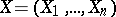, the componentsof which are independent, identically distributed random variables taking values in a sampling space,, it is necessary to estimate the unknown true value of the parameter. Next, suppose that onacts a group of one-to-one transformations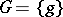such that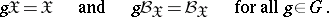In turn, the groupgenerates on the parameter spacea so-called induced group of transformations, the elements of which are defined by the formula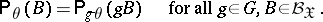Let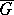be a group of one-to-one transformations onsuch thatUnder these conditions it is said that a point estimatorofis an equivariant estimator, or that it preserves the structure of the problem of statistical estimation of the parameterwith respect to the group, if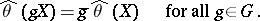The most interesting results in the theory of equivariant estimators have been obtained under the assumption that the loss function is invariant with respect to.

How to Cite This Entry:
Equivariant estimator. Encyclopedia of Mathematics. URL: http://encyclopediaofmath.org/index.php?title=Equivariant_estimator&oldid=15622
This article was adapted from an original article by M.S. Nikulin (originator), which appeared in Encyclopedia of Mathematics - ISBN 1402006098. See original article